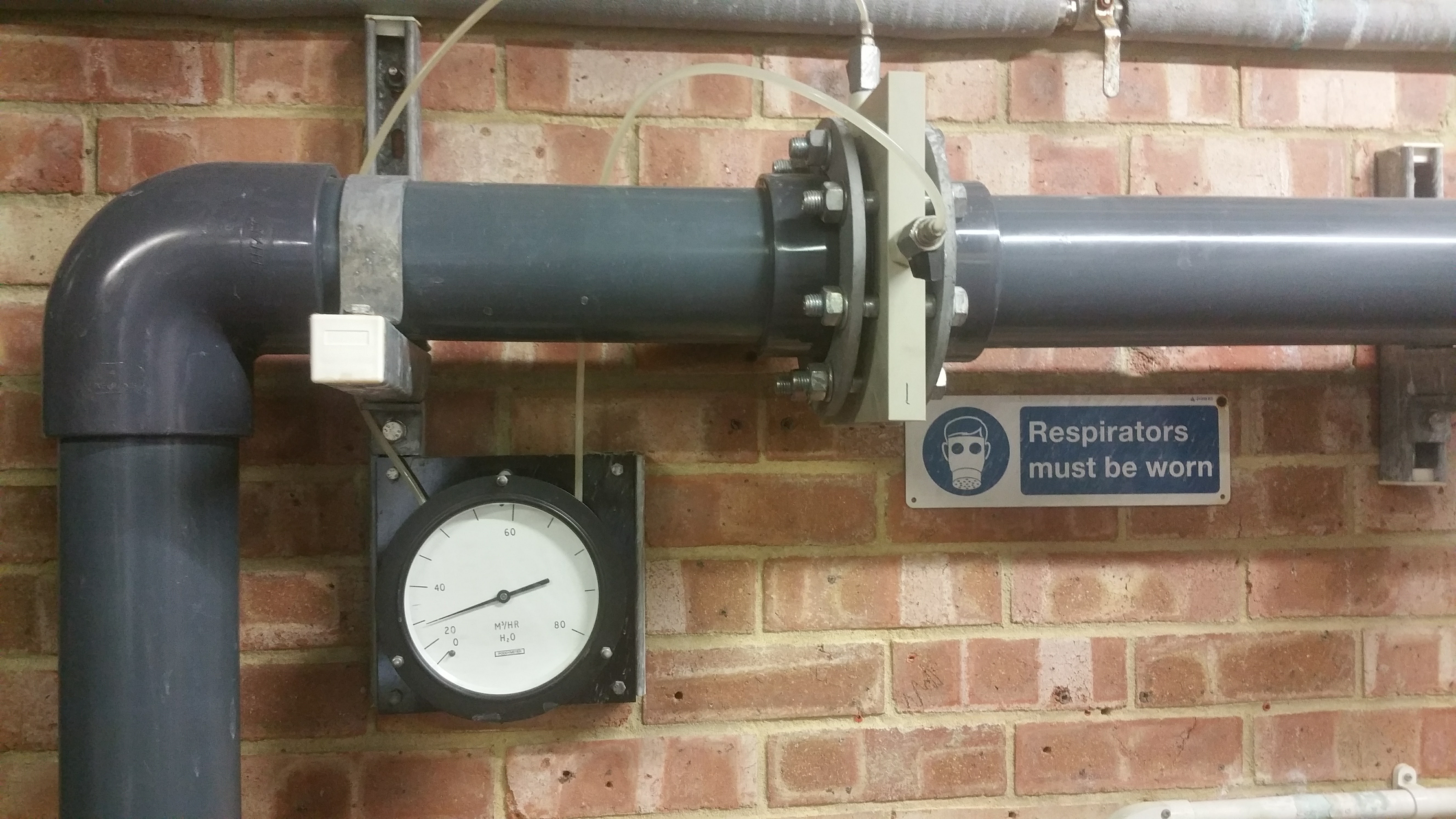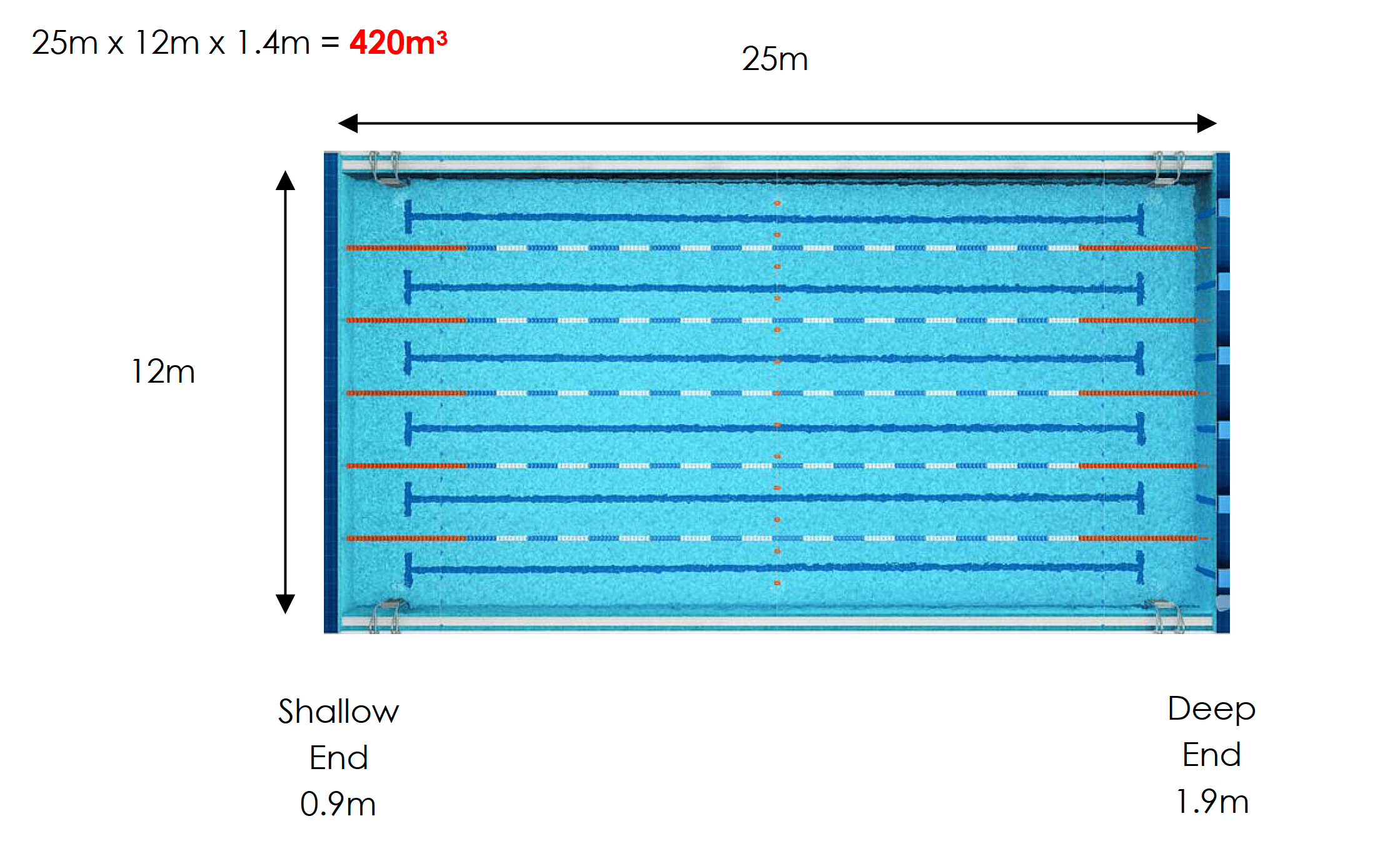# Swimming Pool Turnover Explained### Swimming Pool Turnover

Swimming pool turnover is the time it takes for a volume of water equivalent to the swimming pool volume to make one pass through the system. It is calculated by dividing the volume by the flow rate.

### Recommended Turnover Rates

• Diving Pools 4 – 8 Hours
• Domestic Pools 4 – 8 Hours
• 50m Olympic Pools 3 – 4 Hours
• 25m General Use Pools 2.5 – 3 Hours
• Leisure Pools Over 1.5m Deep 2 – 2.5 Hours
• Leisure Pools 1 – 1.5m Deep 1 – 2 Hours
• Leisure Pools 0.5 – 1m Deep 0.5 – 1.25 Hours
• Leisure Pools Less Than 0.5m Deep 10 – 45 mins
• Hydrotherapy Pools 0.5 – 1.5 Hours
• Teaching Pools 0.5 – 1.5 Hours
• Waterside Splash Pools 0.5 – 1 Hour
• Interactive Water Features 20 mins
• Domestic Spas 15 mins
• Commercial Spas 6 mins
• Leisure Water Bubble Pools 5 – 20 mins

It would be impossible to calculate the turnover time, without knowing the flow rate. Therefore, all pools should ideally be fitted with a flow rate meter.

This flow rate meter displays the information as cubic metres per hour (m3/hr).

This one displays the information as gallons per minute (GPM) and litres per minute (LPM). To convert litres per minute to cubic metres per hour, multiply the litres per minute by 0.06.

If the turnover time of a pool is too long, pollution levels will start to build up. If the turnover time is too short, the water will be travelling too fast through the system and this will have a negative impact on the effectiveness of the filtration system (slower filtration is usually better than faster filtration).

### Flow Rate

Good circulation hydraulics are necessary to ensure that the whole pool is adequately served in terms of both disinfection and removal of pollutants.

Flow rate refers to the speed at the pool water is circulating around the system and is usually expressed as m3/hr, meaning cubic metres per hour but sometimes the flow rate is given as gallons per minute (GPM) or litres per minute (LPM).

Without knowing the flow rate, you will not be able to calculate the turnover time of the pool, which refers to the amount of time it takes for the pool volume to go through the circulation system.

• Flow meters should be maintained and calibrated on a regular basis to ensure that the information they provide is accurate.

• Flow Rate – The speed at which water is travelling through the circulation system, usually expressed as cubic metres per hour (m3/hr).

• Turnover Time – The time it takes for a volume of water equivalent to that of the pool contents to make one pass through the circulation system, usually expressed as hours and/or minutes

• Pool Volume – The volume of the swimming pool, which can be calculated by multiplying the length by the width by the average depth and usually expressed as cubic metres (m3).

For example, if you were operating a swimming pool that is 25m long, 12m wide, with an average depth of 1.4m, the pool volume would be worked out like this:If the flow rate was indicated as being 150m3/hr (from the flow rate meter in the plant room), then the pool turnover would be calculated like this:

420m3 / 150m3/h = 2.8 hours